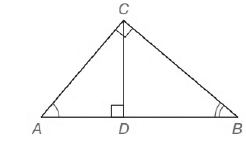Chapter 5.CT, Problem 6CTElementary Geometry For College St...

7th Edition
Alexander + 2 others
ISBN: 9781337614085

Solutions

Chapter
SectionElementary Geometry For College St...

7th Edition
Alexander + 2 others
ISBN: 9781337614085
Textbook Problem

In right triangle A B C ,   C D - is the altitude from C to hypotenuse A B - . Name three triangles that are similar to each other.To determine

To find:

The triangles that is similar to each other.

Explanation

Given,

A right triangle ABC,CD¯ is the altitude from C to hypotenuse AB¯.

Here, the altitude cd¯ is drawn to the hypotenuse of a right triangle ABC that separates the right triangle into two right triangles as ADC,CDB

Therefore, we have the smaller triangles as follows,

Consider the ABC,ACD

Here, AA

BC

CD

AAA Theorem:

If the three angles of one triangle are congruent to the three angles of a second triangle then the triangles are similar.

Therefore by the above theorem

ABC~ACD

Consider the ABC,CBD

Still sussing out bartleby?

Check out a sample textbook solution.

See a sample solution

The Solution to Your Study Problems

Bartleby provides explanations to thousands of textbook problems written by our experts, many with advanced degrees!

Get Started

Find the area of the shaded region.

Single Variable Calculus: Early Transcendentals, Volume I

In Exercises 2336, find the domain of the function. 30. g(x)=2x2+3

Applied Calculus for the Managerial, Life, and Social Sciences: A Brief Approach

Which is the best graph of y = f (x) for the given graph of y = f (x)? a) None of these

Study Guide for Stewart's Single Variable Calculus: Early Transcendentals, 8th

True or False: The function f is continuous at (0, 0), where

Study Guide for Stewart's Multivariable Calculus, 8th